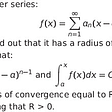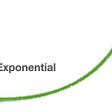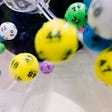# Question:

Three ants are sitting at the three corners of an equilateral triangle. Each ant starts randomly picks a direction and starts to move along the edge of the triangle. What is the probability that none of the ants collide?

# Solution: 0.25

`So let’s think this through. The ants can only avoid a collision if they all decide to move in the same direction (either clockwise or anti-clockwise). If the ants do not pick the same direction, there will definitely be a collision. Each ant has the option to either move clockwise or anti-clockwise. There is a one in two chance that an ant decides to pick a particular direction. Using simple probability calculations, we can determine the probability of no collision.P(No collision) = P(All ants go in a clockwise direction) + P( All ants go in an anti-clockwise direction) = 0.5 * 0.5 * 0.5 + 0.5 * 0.5 * 0.5 = 0.25`

Placewit grows the best engineers by providing an interactive classroom experience and by helping them develop their skills and get placed in amazing companies.

--

--

--

## More from Placewit

Upskilling students for tech placements!

Love podcasts or audiobooks? Learn on the go with our new app.

## Making Calculations with the Power Series## 2020 — The year of Exponential## Introduction to Volatility, Part 2## Differential Equations 10: The Nonhomogeneous Equation & Constant Coefficient Homogeneous Equations## Mathematical induction: What is it, what’s used for, and how to apply it.## Differential Equations 14: The Cauchy-Euler Equation & Variation of Parameters## A Wager, Rolling Restarts and Euler’s Number## An Introduction to Convex Learning Problems## Placewit

Upskilling students for tech placements!

## Ratio of Boys and Girls Puzzle## How to perform range addition in O(n) time and O(1) space complexity?## One interview, Many learnings## Interview preparation -DSA #day1